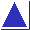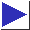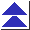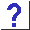# comp.lang.c FAQ list ·Question 20.29

Q: What is hashing?

A: Hashing is the process of mapping strings to integers, usually in a relatively small range. A ``hash function'' maps a string (or some other data structure) to a bounded number (the ``hash bucket'') which can more easily be used as an index in an array, or for performing repeated comparisons. (Obviously, a mapping from a potentially huge set of strings to a small set of integers will not be unique. Any algorithm using hashing therefore has to deal with the possibility of ``collisions.'')

Many hashing functions and related algorithms have been developed; a full treatment is beyond the scope of this list. An extremely simple hash function for strings is simply to add up the values of all the characters:

```unsigned hash(char *str)
{
unsigned int h = 0;
while(*str != '\0')
h += *str++;
return h % NBUCKETS;
}
```
A somewhat better hash function is
```unsigned hash(char *str)
{
unsigned int h = 0;
while(*str != '\0')
h = (256 * h + *str++) % NBUCKETS;
return h;
}
```
which actually treats the input string as a large binary number (8 * strlen(str) bits long, assuming characters are 8 bits) and computes that number modulo NBUCKETS, by Horner's rule. (Here it is important that NBUCKETS be prime, among other things. To remove the assumption that characters are 8 bits, use UCHAR_MAX+1 instead of 256; the ``large binary number'' will then be CHAR_BIT * strlen(str) bits long. UCHAR_MAX and CHAR_BIT are defined in <limits.h>.)

When the set of strings is known in advance, it is also possible to devise ``perfect'' hashing functions which guarantee a collisionless, dense mapping.

References: K&R2 Sec. 6.6
Knuth Sec. 6.4 pp. 506-549 Volume 3
Sedgewick Sec. 16 pp. 231-244